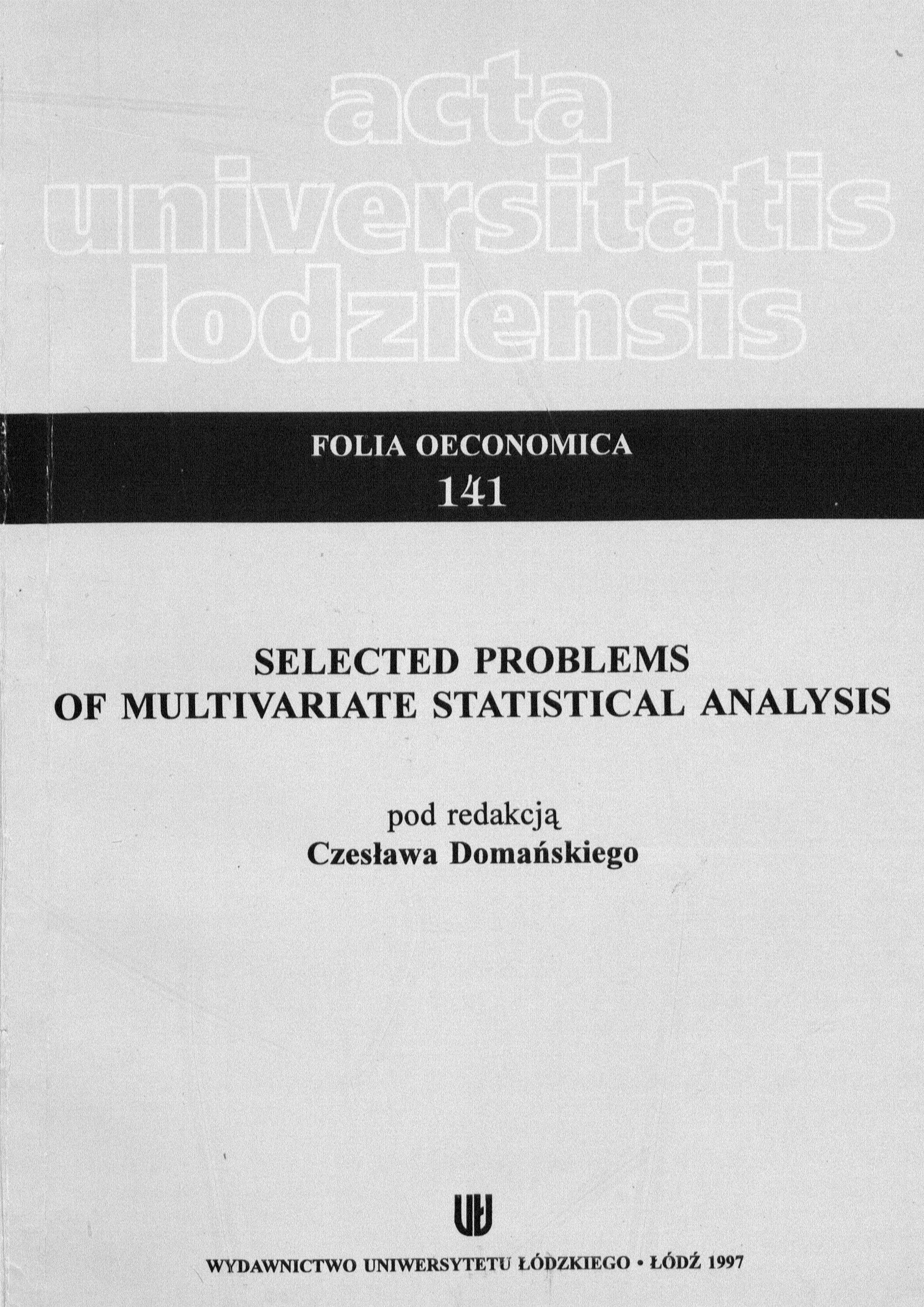### Browse byCONTENT

Zadanie pt. Digitalizacja i udostępnienie w Cyfrowym Repozytorium Uniwersytetu Łódzkiego kolekcji czasopism naukowych wydawanych przez Uniwersytet Łódzki nr 885/P-DUN/2014 zostało dofinansowane ze środków MNiSW w ramach działalności upowszechniającej naukę.

### Recent Submissions

• #### Limit laws for multivalued random variables ﻿

(Wydawnictwo Uniwersytetu Łódzkiego, 1997)
In the probability theory, the strong law of large numbers and the central limit theorem are the most important convergence theorems.
• #### A Monte Carlo investigation of two distance measures between statistical populations and their application to cluster analysis ﻿

(Wydawnictwo Uniwersytetu Łódzkiego, 1997)
The paper deals with a simulation study of one of the well-known hierarchical cluster analysis methods applied to classifying the statistical populations. In particular, the problem of clustering the univariate normal ...
• #### Decomposition of time series on the basis of modified grouping method of Ward ﻿

(Wydawnictwo Uniwersytetu Łódzkiego, 1997)
The trend of time series can change its direction. It is assumed that the time interval is divided into subintervals where the trend is given as particular linear function. The problem is how to divide the observation ...
• #### Application of the sequential probability ratio test to verification of statistical hypotheses ﻿

(Wydawnictwo Uniwersytetu Łódzkiego, 1997)
The paper deals with some problems concerning the sequential probability ratio tests (SPRT) and their application to verifying simple and composite statistical hypotheses. Besides properties and examples of SPRT, there ...
• #### Characterization o f the orders in VARMA models ﻿

(Wydawnictwo Uniwersytetu Łódzkiego, 1997)
In tins paper we present a method which is able to identify the degrees of the matrix polynomials that are involved in the VARMA models. This method is based on the difference between the ranks of certain matrices defined ...
• #### Switching regression models with non-normal errors ﻿

(Wydawnictwo Uniwersytetu Łódzkiego, 1997)
In this paper two forms of switching regression models with non-normal errors are considered. The pseudo maximum likelihood method is proposed for the estimation of their parameters. Monte Carlo experiments results are ...
• #### On the classification of observations in the switching regression ﻿

(Wydawnictwo Uniwersytetu Łódzkiego, 1997)
The paper discusses the method of determining the sample division indicator for the switching regression model in case of two states generating values of the explained variable, which ensures the least risk of making a ...
• #### Bayesian estimation of a shift point in a two-phase regression model ﻿

(Wydawnictwo Uniwersytetu Łódzkiego, 1997)
The purpose of this paper is to carry out the Bayesian analysis of a two-phase regression model with an unknown break point. Essentially, there are two problems associated with a changing linear model. Firstly, one will ...
• #### The distribution and parameters of the distribution of the quotient of random variables ﻿

(Wydawnictwo Uniwersytetu Łódzkiego, 1997)
This paper presents some important earlier results of the research concerning the properties of the distribution of the quotient of random variables. We present also our own ideas and results of the research concerning ...
• #### Application of Dagum economic distances to the analysis of wage distributions in Poland ﻿

(Wydawnictwo Uniwersytetu Łódzkiego, 1997)
Studies in the field of wage and income distribution concentrate on measuring income inequality. We can investigate both the inequality within the population and the inequality between the populations. The latter can be ...
• #### Asymptotic results for sliced inverse regression ﻿

(Wydawnictwo Uniwersytetu Łódzkiego, 1997)
It is well known that nonparametric regression techniques do not have good performance in high dimensional regression. However nonparametric regression is successful in one- or low-dimensional regression problems and is ...
• #### Usefullness of Durbin method for investigating normality in one-dimensional linear model ﻿

(Wydawnictwo Uniwersytetu Łódzkiego, 1997)
In this paper the verification of hypotheses of univariate normality by Durbin randomized method is presented. The method of elimination of the nuisance parameters by calculating the residual vector and connected residual ...
• #### Remarks on some learning algorithms ﻿

(Wydawnictwo Uniwersytetu Łódzkiego, 1997)
In this paper we present some problems concerning artificial intelligence. In the first two points there are presented algorithmic and heuristic procedures, which are applied in solving problems and making optimal decision ...
• #### Preface ﻿

(Wydawnictwo Uniwersytetu Łódzkiego, 1997)
• #### The verification of the multivariate normal distribution hypothesis in a one-sample model with the method of elimination of disturbing parameters ﻿

(Wydawnictwo Uniwersytetu Łódzkiego, 1997)
In many statistical tasks a necessity of testing multivariate normality arises. In constructing multivariate normality tests there is a necessity of estimating unknown parameters ц and £ from a given sample. The parameters ...
• #### Remarks on the generalized doubly truncated gamma distribution ﻿

(Wydawnictwo Uniwersytetu Łódzkiego, 1997)
In the present paper we discuss a doubly truncated generalized gamma distribution and give formulae for the moments of this distribution and special cases together with examples of calculations.